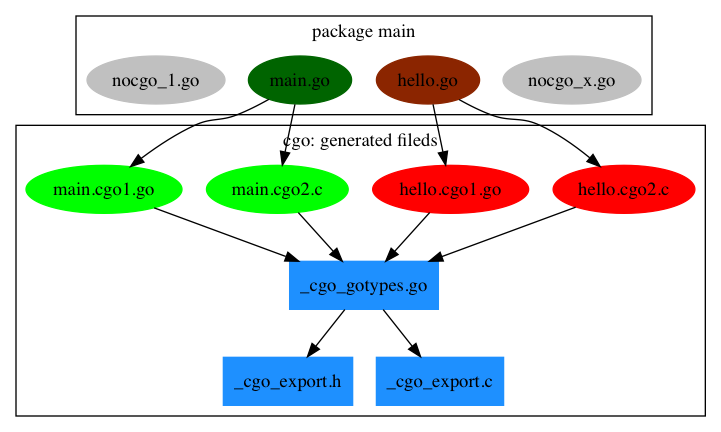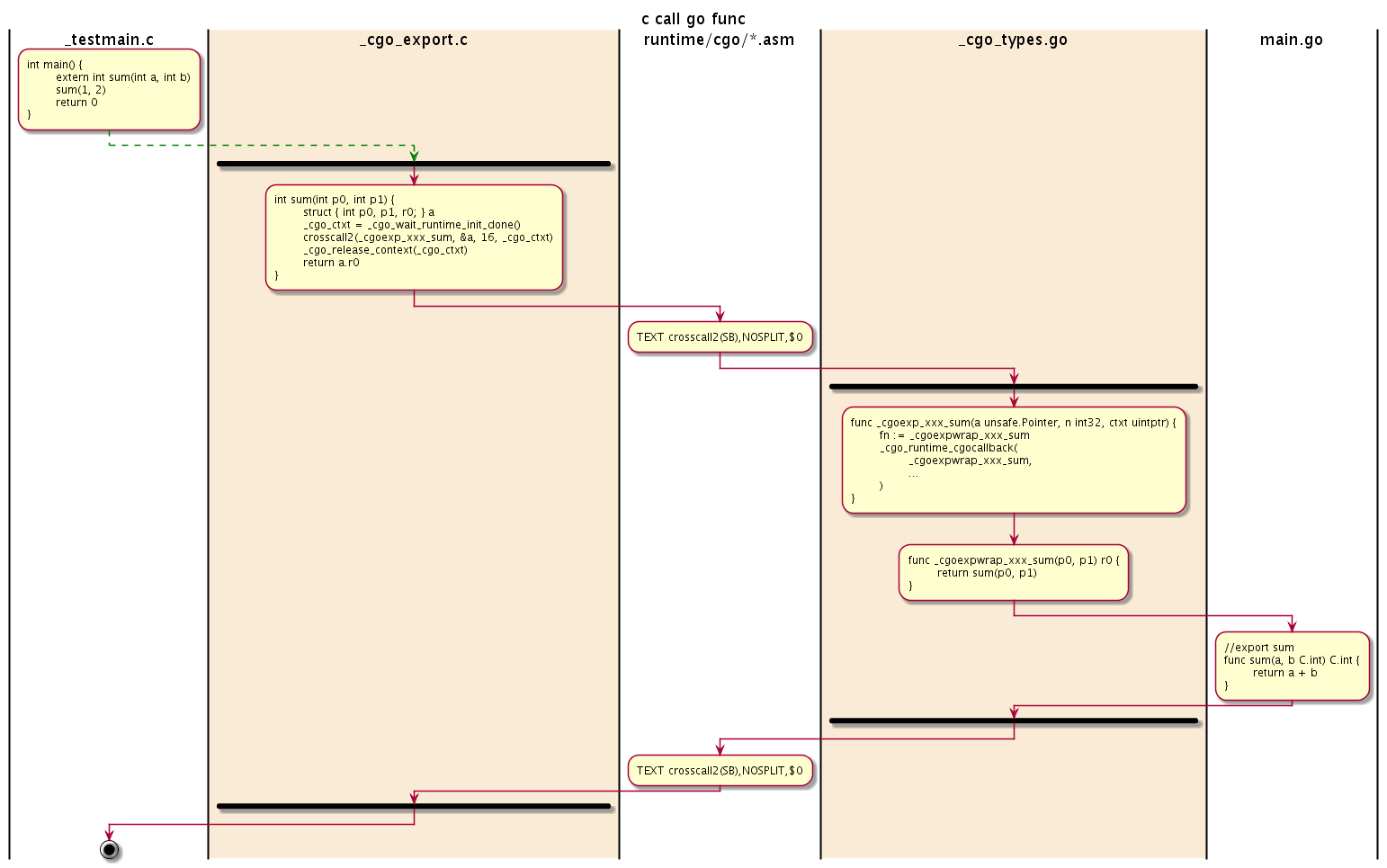# 2.5 内部机制

## 2.5.1 CGO生成的中间文件## 2.5.2 Go调用C函数

Go调用C函数是CGO最常见的应用场景，我们将从最简单的例子入手分析Go调用C函数的详细流程。

``````package main

//int sum(int a, int b) { return a+b; }
import "C"

func main() {
println(C.sum(1, 1))
}
``````

``````\$ go tool cgo main.go
``````

``````\$ ls _obj | awk '{print \$NF}'
_cgo_.o
_cgo_export.c
_cgo_export.h
_cgo_flags
_cgo_gotypes.go
_cgo_main.c
main.cgo1.go
main.cgo2.c
``````

``````package main

//int sum(int a, int b) { return a+b; }
import _ "unsafe"

func main() {
println((_Cfunc_sum)(1, 1))
}
``````

`_Cfunc_sum`函数在cgo生成的`_cgo_gotypes.go`文件中定义：

``````//go:cgo_unsafe_args
func _Cfunc_sum(p0 _Ctype_int, p1 _Ctype_int) (r1 _Ctype_int) {
_cgo_runtime_cgocall(_cgo_506f45f9fa85_Cfunc_sum, uintptr(unsafe.Pointer(&p0)))
if _Cgo_always_false {
_Cgo_use(p0)
_Cgo_use(p1)
}
return
}
``````

`_Cfunc_sum`函数的参数和返回值`_Ctype_int`类型对应`C.int`类型，命名的规则和`_Cfunc_xxx`类似，不同的前缀用于区分函数和类型。

``````func runtime.cgocall(fn, arg unsafe.Pointer) int32
``````

``````void _cgo_506f45f9fa85_Cfunc_sum(void *v) {
struct {
int p0;
int p1;
int r;
} __attribute__((__packed__)) *a = v;
char *stktop = _cgo_topofstack();
__typeof__(a->r) r;
_cgo_tsan_acquire();
r = sum(a->p0, a->p1);
_cgo_tsan_release();
a = (void*)((char*)a + (_cgo_topofstack() - stktop));
a->r = r;
}
``````

`_cgo_506f45f9fa85_Cfunc_sum`函数的指针指向的结构为：

``````    struct {
int p0;
int p1;
int r;
} __attribute__((__packed__)) *a = v;
``````

`C.sum`的整个调用流程图如下：## 2.5.3 C调用Go函数

``````package main

//int sum(int a, int b);
import "C"

//export sum
func sum(a, b C.int) C.int {
return a + b
}

func main() {}
``````

CGO的语法细节不在赘述。为了在C语言中使用sum函数，我们需要将Go代码编译为一个C静态库：

``````\$ go build -buildmode=c-archive -o sum.a sum.go
``````

``````\$ go tool cgo main.go
``````

_obj目录还是生成类似的中间文件。为了查看方便，我们刻意忽略了无关的几个文件：

``````\$ ls _obj | awk '{print \$NF}'
_cgo_export.c
_cgo_export.h
_cgo_gotypes.go
main.cgo1.go
main.cgo2.c
``````

``````int sum(int p0, int p1)
{
__SIZE_TYPE__ _cgo_ctxt = _cgo_wait_runtime_init_done();
struct {
int p0;
int p1;
int r0;
} __attribute__((__packed__)) a;
a.p0 = p0;
a.p1 = p1;
_cgo_tsan_release();
crosscall2(_cgoexp_8313eaf44386_sum, &a, 16, _cgo_ctxt);
_cgo_tsan_acquire();
_cgo_release_context(_cgo_ctxt);
return a.r0;
}
``````

sum函数的内容采用和前面类似的技术，将sum函数的参数和返回值打包到一个结构体中，然后通过`runtime/cgo.crosscall2`函数将结构体传给`_cgoexp_8313eaf44386_sum`函数执行。

`runtime/cgo.crosscall2`函数采用汇编语言实现，它对应的函数声明如下：

``````func runtime/cgo.crosscall2(
fn func(a unsafe.Pointer, n int32, ctxt uintptr),
a unsafe.Pointer, n int32,
ctxt uintptr,
)
``````

``````func _cgoexp_8313eaf44386_sum(a unsafe.Pointer, n int32, ctxt uintptr) {
fn := _cgoexpwrap_8313eaf44386_sum
_cgo_runtime_cgocallback(**(**unsafe.Pointer)(unsafe.Pointer(&fn)), a, uintptr(n), ctxt);
}

func _cgoexpwrap_8313eaf44386_sum(p0 _Ctype_int, p1 _Ctype_int) (r0 _Ctype_int) {
return sum(p0, p1)
}
``````

`_cgo_runtime_cgocallback`函数对应`runtime.cgocallback`函数，函数的类型如下：

``````func runtime.cgocallback(fn, frame unsafe.Pointer, framesize, ctxt uintptr)
``````